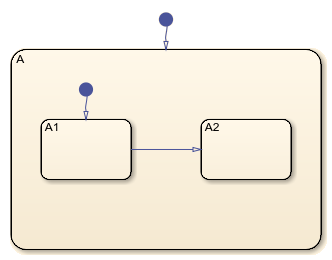## Access Objects in Your Stateflow Chart

The objects in the Stateflow® API represent the graphical and nongraphical objects of a Stateflow chart. For example, the API objects `Stateflow.State` and `Stateflow.Transition` represent states and transitions in a Stateflow chart. For more information, see Overview of the Stateflow API.

### Find Objects in a Chart

With the `find` function, you can locate an API object by specifying search criteria. You can combine criteria such as:

• The type of object

• The name of a property or function

• A property name and value

For example, this command searches the `Simulink.Root` object and returns every `Stateflow.State` object with the name `On`:

`onState = find(sfroot,"-isa","Stateflow.State",Name="On")`

If more than one object meets the search criteria, `find` returns an array of qualifying objects. For example, if more than one chart is open, this command returns an array of `Stateflow.Chart` objects:

`chartArray = find(sfroot,"-isa","Stateflow.Chart")`

#### Find Objects at Specific Levels of Containment

By default, the `find` function finds objects at all depths of containment within an object. For example, suppose that `ch` is a `Stateflow.Chart` object that corresponds to this chart. The chart contains a parent state `A` with two child states, `A1` and `A2`. For more information on this example, see Create Charts by Using a MATLAB Script.Calling the `find` function to find all the states in this chart returns an array with three `Stateflow.State` objects:

```states = find(ch,"-isa","Stateflow.State"); get(states,"Name")```
```ans = 3×1 cell array {'A'} {'A1'} {'A2'}```

To limit the maximum containment depth of a search, use the `"-depth"` argument as part of your search criteria. For example, to find the only `Stateflow.State` object at the first level of containment in `ch`, enter:

```sA = find(ch,"-isa","Stateflow.State","-depth",1); sA.Name```
```ans = 'A'```

Similarly, you can call the `find` function to search for states in the first level of containment in the `Stateflow.State` object `sA`. In this case, the search includes the zeroth level of containment, which is the searched object itself:

```states = find(sA,"-isa","Stateflow.State","-depth",1); get(states,"Name")```
```ans = 3×1 cell array {'A'} {'A1'} {'A2'}```

To exclude state `A` from the search results, call the MATLAB® function `setdiff`:

```childStates = setdiff(states,sA); get(childStates,"Name")```
```ans = 2×1 cell array {'A1'} {'A2'}```

### Navigate the Stateflow Hierarchy

After you access an API object, you can use the `getChildren` and `getParent` functions to navigate through the Stateflow hierarchy and identify the children that the object contains or the parent that contains the object.

#### Find Child Objects

To find the children of an API object, call the `getChildren` function. For instance, suppose that `ch` is the `Stateflow.Chart` object that corresponds to the chart in the previous example. Calling the `getChildren` function on `ch` returns an array that contains a `Stateflow.State` object and a `Stateflow.Transition` object.

```children = getChildren(ch); arrayfun(@class,children,UniformOutput=false)```
```ans = 2×1 cell array {'Stateflow.State' } {'Stateflow.Transition'}```

The first element in the array is a `Stateflow.State` object that corresponds to state `A`.

```state = children(1); state.Name```
```ans = 'A'```

The second element in the array is a `Stateflow.Transition` object that corresponds to the default transition into state `A`.

`children(2).Source`
```ans = []```
`children(2).Destination.Name`
```ans = 'A'```

Similarly, calling the `getChildren` function on the state returns an array that contains two `Stateflow.State` objects and two `Stateflow.Transition` objects.

```grandchildren = getChildren(state); arrayfun(@class,grandchildren,UniformOutput=false)```
```ans = 4×1 cell array {'Stateflow.State' } {'Stateflow.State' } {'Stateflow.Transition'} {'Stateflow.Transition'}```

The first and second elements in this array are `Stateflow.State` objects that correspond to the states `A1` and `A2`.

`grandchildren(1).Name`
```ans = 'A1'```
`grandchildren(2).Name`
```ans = 'A2'```

The third and fourth elements in `grandchildren` are `Stateflow.Transition` objects that correspond to the transitions into states `A1` and between state `A1` and `A2`, respectively.

`grandchildren(3).Source`
```ans = []```
`grandchildren(3).Destination.Name`
```ans = 'A1'```
`grandchildren(4).Source.Name`
```ans = 'A1'```
`grandchildren(4).Destination.Name`
```ans = 'A2'```

#### Find Parent Object

To find the parent of an API object, call the `getParent` function. For instance, suppose that `sA1` is the `Stateflow.State` object that corresponds to state `A1` in the previous example. Calling the `getParent` function on `sA1` returns the `Stateflow.State` object that corresponds to state `A`:

```parent = getParent(sA1); parent.Name```
```ans = 'A'```

Similarly, calling the `getParent` function on `parent` returns the `Stateflow.Chart` object that corresponds to the chart:

```grandparent = getParent(parent); grandparent.Name```
```ans = 'Chart' ```

### Retrieve Recently Selected Objects

You can retrieve the most recently selected objects in a chart by calling the `sfgco` function. This function returns a single object or an array of objects, depending on your selection.

For instance, suppose that you select the transition from state `A1` to state `A2` in the previous example. Calling `sfgco` returns the corresponding `Stateflow.Transition` object:

```tr = sfgco; str = str = "Transition from "+tr.Source.Name+" to "+tr.Destination.Name```
```str = "Transition from A1 to A2"```

Similarly, if you simultaneously select the three states in the chart, calling `sfgco` returns an array of `Stateflow.State` objects.

```states = sfgco; get(states,"Name")```
```ans = 3×1 cell array {'A'} {'A1'} {'A2'}```

Note

When you use `sfgco` to access multiple objects, the order of the objects in the array depends on the order in which you select the objects.